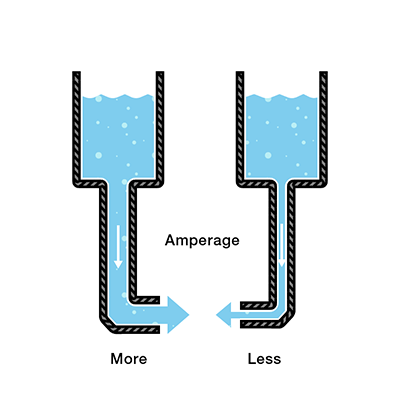# Intro to Electronics — Day 1

## Each Group Collect

• Digital Multimeter
• Variable Voltage Power Supply
• Resistors
• DC Motor

## What is Electricity?

A general understanding of electricity and the rules that determine how it flows and how it can be controlled are crucial to quickly prototyping your designs. These resources cover the fundamentals of working with electricity.

### Water analogy from SparkfunVoltage = Pressure in systemCurrent (Amperage) = Amount of FlowResistance = Resistance to FlowV = I * R (voltage = current * resistance)

I = V / R (current = voltage / resistance)

## Solve for LED / Resistor Combo

We know the ideal forward voltage (Vf) and current (I) of the LED.

Target Vf = 3.3v

Target I = 20mA (0.02A)

V = I * R

R = (Vs – Vf) / I

### Exercise 1: Learn the Digital Multimeter (DMM)

Sparkfun: How to Use a Multimeter

1. Set up a simple circuit on a breadboard with an LED, a resistor, and the variable voltage power supply:
1. Anode (+) [because Node.js is positive/great]
2. Cathode (-) [because cats are negative/terrible]
3.2. Set the power supply to 5V
3. Use the the DMM to measure voltage at different points of the circuit
4. Notice the amount of current being used by the power supply
5. Insert the DMM into the circuit and measure the amount of current (see if it matches what the power supply says)
6. What happens if you change the resistor?
7. Add a switch or button
8. Draw the [circuit diagram](https://learn.sparkfun.com/tutorials/how-to-read-a-schematic) on the table

## What is Arduino?

A simple microcontroller and software combo developed to help designers, artists and non-engineers rapidly prototype with electronics.

#### Some things to remember:

1. Analog Pins are for reading only (0v – 5v)
2. `analogRead(pinNumber)` returns an integer value between 0 – 1023
3. Digital Pins can be `INPUT` or `OUTPUT`
1. digital pins can supply a low-current (100mA) 5v supply in output mode:  `digitalWrite(pin, HIGH/LOW);`
2. digital pins can read the state of that pin, as either `HIGH` or `LOW` (5v or 0v): `digitalRead(pin);`
4. You can run the arduino WITHOUT the USB cable attached (only a 5v-24v barrel jack)
1. You can also power from the Vin and GND pins.

### Exercise 2: Set up Arduino & Blink

2. Follow the Getting Started guide for the Arduino UNO (word for word!)
3. Run the Blink! example sketch
1. Open Examples > Basics > Blink
2. Plug your Arduino into the computer
4. Choose the correct port: Tools>Port
5. Click the check icon (verify)
6. If no errors, upload to the arduino

### Exercise 3: Reading Data & Graphing it

1. Find a sensor (Potentiometers are a good one)
2. Attach it to the Analog pins of your Arduino
• You may need to find a diagram/datasheet/instructions on the internet
3. Graph the output in either Max or Processing (or both!)